### I just announced the new Learn Spring course, focused on the fundamentals of Spring 5 and Spring Boot 2:

>> CHECK OUT THE COURSE

## 1. Overview

In this tutorial, we'll understand the basic concepts of a graph as a data structure.

We'll also explore its implementation in Java along with various operations possible on a graph. We will also discuss the Java libraries offering graph implementations.

## Checking if a Java Graph has a Cycle

Learn how to check if a cycle exists in a given directed graph in Java.

## Dijkstra Shortest Path Algorithm in Java

An explanation and implementation of the Dijkstra Algorithm in Java

## Introduction to JGraphT

Learn how to use JGraphT to create graphs and explore the variety of graph algorithms.

## 2. Graph Data Structure

A graph is a data structure for storing connected data like a network of people on a social media platform.

A graph consists of vertices and edges. A vertex represents the entity (for example, people) and an edge represents the relationship between entities (for example, a person's friendships).

Let's define a simple Graph to understand this better: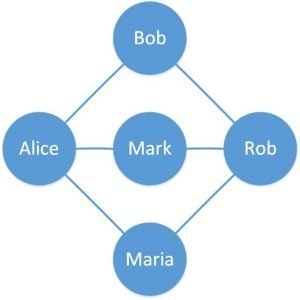Here, we've defined a simple graph with five vertices and six edges. The circles are vertices representing people and the lines connecting two vertices are edges representing friends on an online portal.

There are a few variations of this simple graph depending on the properties of the edges. Let's briefly go through them in the next sections.

However, we'll only focus on the simple graph presented here for the Java examples in this tutorial.

### 2.1. Directed Graph

The graph we've defined so far has edges without any direction. If these edges feature a direction in them, the resulting graph is known as a directed graph.

An example of this can be representing who send the friend request in a friendship on the online portal: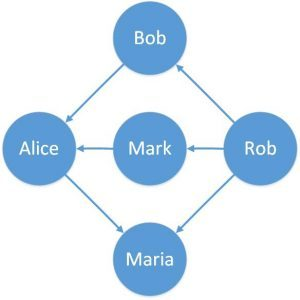Here, we can see that the edges have a fixed direction. The edges can be bi-directional as well.

### 2.2. Weighted Graph

Again, our simple graph has edges which are unbiased or unweighted. If instead these edges carry relative weight, this graph is known as a weighted graph.

An example of a practical application of this can be representing how relatively old is a friendship on the online portal: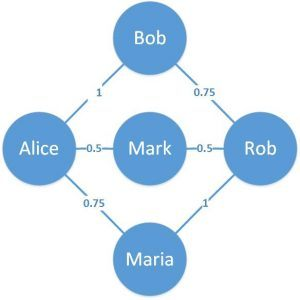Here, we can see that the edges have weights associated with them. This provides a relative meaning to these edges.

## 3. Graph Representations

A graph can be represented in different forms like adjacency matrix and adjacency list. Each one has their pros and cons in a different set-up.

We'll introduce these graph representations in this section.

An adjacency matrix is a square matrix with dimensions equivalent to the number of vertices in the graph.

The elements of the matrix typically have values ‘0' or ‘1'. A value of ‘1' indicates adjacency between the vertices in the row and column and a value of ‘0' otherwise.

Let's see how the adjacency matrix looks like for our simple graph from the previous section: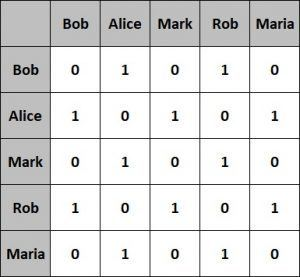This representation is fairly easier to implement and efficient to query as well. However, it's less efficient with respect to space occupied.

An adjacency list is nothing but an array of lists. The size of the array is equivalent to the number of vertices in the graph.

The list at a specific index of the array represents the adjacent vertices of the vertex represented by that array index.

Let's see what the adjacency list looks like for our simple graph from the previous section: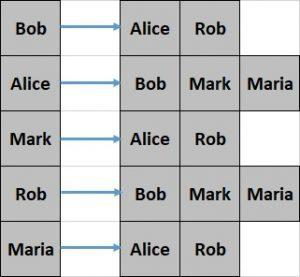This representation is comparatively difficult to create and less efficient to query. However, it offers better space efficiency.

We'll use the adjacency list to represent the graph in this tutorial.

## 4. Graphs in Java

Java doesn't have a default implementation of the graph data structure.

However, we can implement the graph using Java Collections.

Let's begin by defining a vertex:

``````class Vertex {
String label;
Vertex(String label) {
this.label = label;
}

// equals and hashCode
}``````

The above definition of vertex just features a label but this can represent any possible entity like Person or City.

Also, note that we have to override the equals() and hashCode() methods as these are necessary to work with Java Collections.

As we discussed earlier, a graph is nothing but a collection of vertices and edges which can be represented as either an adjacency matrix or an adjacency list.

Let's see how we can define this using an adjacency list here:

``````class Graph {

// standard constructor, getters, setters
}``````

As we can see here, the class Graph is using Map from Java Collections to define the adjacency list.

There are several operations possible on a graph data structure, such as creating, updating or searching through the graph.

We'll go through some of the more common operations and see how we can implement them in Java.

## 5. Graph Mutation Operations

To start with, we'll define some methods to mutate the graph data structure.

Let's define methods to add and remove vertices:

``````void addVertex(String label) {
}

void removeVertex(String label) {
Vertex v = new Vertex(label);
}``````

These methods simply add and remove elements from the vertices Set.

Now, let's also define a method to add an edge:

``````void addEdge(String label1, String label2) {
Vertex v1 = new Vertex(label1);
Vertex v2 = new Vertex(label2);
}``````

This method creates a new Edge and updates the adjacent vertices Map.

In a similar way, we'll define the removeEdge() method:

``````void removeEdge(String label1, String label2) {
Vertex v1 = new Vertex(label1);
Vertex v2 = new Vertex(label2);
if (eV1 != null)
eV1.remove(v2);
if (eV2 != null)
eV2.remove(v1);
}``````

Next, let's see how we can create the simple graph we have drawn earlier using the methods we've defined so far:

``````Graph createGraph() {
Graph graph = new Graph();
return graph;
}``````

We'll finally define a method to get the adjacent vertices of a particular vertex:

``````List<Vertex> getAdjVertices(String label) {
}``````

## 6. Traversing a Graph

Now that we have graph data structure and functions to create and update it defined, we can define some additional functions for traversing the graph. We need to traverse a graph to perform any meaningful action, like search within the graph.

There are two possible ways to traverse a graph, depth-first traversal and breadth-first traversal.

### 6.1. Depth-First Traversal

A depth-first traversal starts at an arbitrary root vertex and explores vertices as deeper as possible along each branch before exploring vertices at the same level.

Let's define a method to perform the depth-first traversal:

``````Set<String> depthFirstTraversal(Graph graph, String root) {
Stack<String> stack = new Stack<String>();
stack.push(root);
while (!stack.isEmpty()) {
String vertex = stack.pop();
if (!visited.contains(vertex)) {
for (Vertex v : graph.getAdjVertices(vertex)) {
stack.push(v.label);
}
}
}
return visited;
}``````

Here, we're using a Stack to store the vertices that need to be traversed.

Let's run this on the graph we created in the previous subsection:

``assertEquals("[Bob, Rob, Maria, Alice, Mark]", depthFirstTraversal(graph, "Bob").toString());``

Please note that we're using vertex “Bob” as our root for traversal here, but this can be any other vertex.

Comparatively, a breadth-first traversal starts at an arbitrary root vertex and explores all neighboring vertices at the same level before going deeper in the graph.

Now let's define a method to perform the breadth-first traversal:

``````Set<String> breadthFirstTraversal(Graph graph, String root) {
while (!queue.isEmpty()) {
String vertex = queue.poll();
for (Vertex v : graph.getAdjVertices(vertex)) {
if (!visited.contains(v.label)) {
}
}
}
return visited;
}``````

Note that a breadth-first traversal makes use of Queue to store the vertices which need to be traversed.

Let's again run this traversal on the same graph:

``````assertEquals(
"[Bob, Alice, Rob, Mark, Maria]", breadthFirstTraversal(graph, "Bob").toString());``````

Again, the root vertex which is “Bob” here can as well be any other vertex.

## 7. Java Libraries for Graphs

It's not necessary to always implement the graph from scratch in Java. There are several open source and mature libraries available which offers graph implementations.

In the next few subsections, we'll go through some of these libraries.

### 7.1. JGraphT

JGraphT is one of the most popular libraries in Java for the graph data structure. It allows the creation of a simple graph, directed graph, weighted graph, amongst others.

Additionally, it offers many possible algorithms on the graph data structure. One of our previous tutorials covers JGraphT in much more detail.

Google Guava is a set of Java libraries that offer a range of functions including graph data structure and its algorithms.

It supports creating simple Graph, ValueGraph, and Network. These can be defined as Mutable or Immutable.

### 7.3. Apache Commons

Apache Commons is an Apache project that offers reusable Java components. This includes Commons Graph which offers a toolkit to create and manage graph data structure. This also provides common graph algorithms to operate on the data structure.

### 7.4. Sourceforge JUNG

Java Universal Network/Graph (JUNG) is a Java framework that provides extensible language for modeling, analysis, and visualization of any data that can be represented as a graph.

JUNG supports a number of algorithms which includes routines like clustering, decomposition, and optimization.

These libraries provide a number of implementations based on the graph data structure. There are also more powerful frameworks based on graphs, such as Apache Giraph, currently used at Facebook to analyze the graph formed by their users, and Apache TinkerPop, commonly used on top of graph databases.

## 8. Conclusion

In this article, we discussed the graph as a data structure along with its representations. We defined a very simple graph in Java using Java Collections and also defined common traversals for the graph.

We also talked briefly about various libraries available in Java outside the Java platform which provides graph implementations.

As always, the code for the examples is available over on GitHub.

### I just announced the new Learn Spring course, focused on the fundamentals of Spring 5 and Spring Boot 2:

>> CHECK OUT THE COURSE
Inline FeedbacksLaurent Querel
1 year ago

You should add Apache TinkerPop/Gremlin to your list (http://tinkerpop.apache.org/). It’s probably the most widely used java graph library on top of most of graph databases.

1 year ago